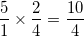# ISEE Lower Level Quantitative : Operations with fractions and whole numbers

## Example Questions

### Example Question #11 : Multiply A Fraction Or Whole Number By A Fraction: Ccss.Math.Content.5.Nf.B.4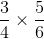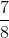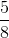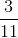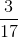Explanation:

When we multiply fractions, we multiply the numerator by the numerator and the denominator by the denominator.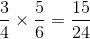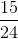can be reduced by dividing both sides by.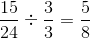### Example Question #12 : Multiply A Fraction Or Whole Number By A Fraction: Ccss.Math.Content.5.Nf.B.4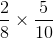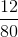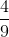Explanation:

When we multiply fractions, we multiply the numerator by the numerator and the denominator by the denominator.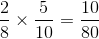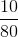can be reduced by dividing each side by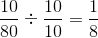### Example Question #13 : Multiply A Fraction Or Whole Number By A Fraction: Ccss.Math.Content.5.Nf.B.4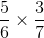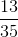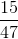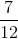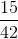Explanation:

When we multiply fractions, we multiply the numerator by the numerator and the denominator by the denominator.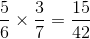### Example Question #14 : Multiply A Fraction Or Whole Number By A Fraction: Ccss.Math.Content.5.Nf.B.4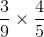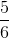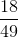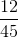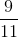Explanation:

When we multiply fractions, we multiply the numerator by the numerator and the denominator by the denominator.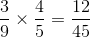### Example Question #15 : Multiply A Fraction Or Whole Number By A Fraction: Ccss.Math.Content.5.Nf.B.4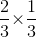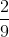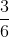Explanation:

When we multiply fractions, we multiply the numerator by the numerator and the denominator by the denominator.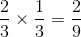### Example Question #1683 : Ssat Middle Level Quantitative (Math)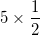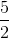Explanation:When we multiply a fraction by a whole number, we first want to make the whole number into a fraction. We do that by putting the whole number over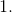The we multiply like normal.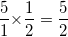### Example Question #1684 : Ssat Middle Level Quantitative (Math)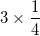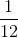Explanation:When we multiply a fraction by a whole number, we first want to make the whole number into a fraction. We do that by putting the whole number overThen we multiply like normal.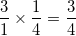### Example Question #1685 : Ssat Middle Level Quantitative (Math)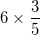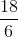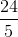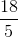Explanation:When we multiply a fraction by a whole number, we first want to make the whole number into a fraction. We do that by putting the whole number overThen we multiply like normal.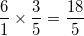### Example Question #818 : Fractions

Solve the following: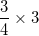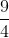Explanation:When we multiply a fraction by a whole number, we first want to make the whole number into a fraction. We do that by putting the whole number overThen we multiply like normal.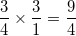### Example Question #1686 : Ssat Middle Level Quantitative (Math)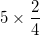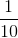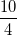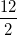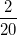When we multiply a fraction by a whole number, we first want to make the whole number into a fraction. We do that by putting the whole number overThen we multiply like normal.